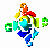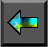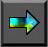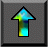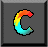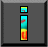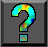Next: 11.1 The Hypercube Template Up: Part III: Resources Previous: Chapter Notes

# 11 Hypercube Algorithms

In Chapter 2, we pointed out that the communication requirements of a reduction operation can be structured as a series of pairwise exchanges, one with each neighbor in a hypercube (butterfly) structure. This structure allows a computation requiring all-to-all communication among P tasks to be performed in just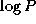steps, rather than P steps as might be expected from a superficial analysis.

It turns out that the hypercube structure can be used to implement   many other parallel algorithms requiring all-to-all communication; that is, algorithms in which each task must communicate with every other task. In this chapter, we review three such algorithms: vector reduction, matrix transposition, and sorting. The purpose of this   discussion is both to describe some useful algorithms and to introduce   the concept   of a parallel algorithm template. A template is a basic program form that a programmer can augment with application-specific information to implement a particular parallel algorithm. The hypercube communication structure described in this chapter is one of the most useful templates in parallel computing.

After studying this chapter, you should have a good understanding of the hypercube communication structure and how it is used to implement all-to-all communication in parallel algorithms. You should also be familiar with the concept of a template and the role templates play in parallel algorithm design and programming.Next: 11.1 The Hypercube Template Up: Part III: Resources Previous: Chapter Notes